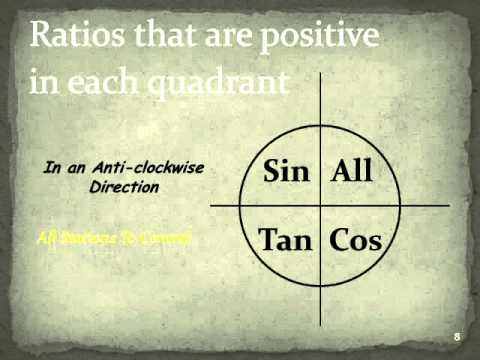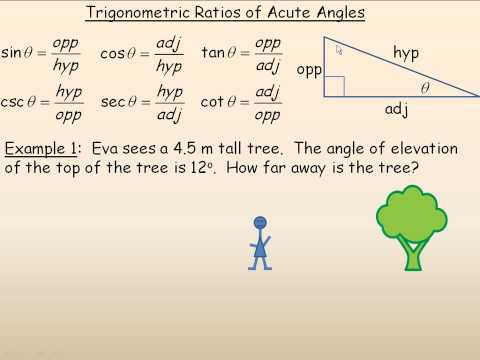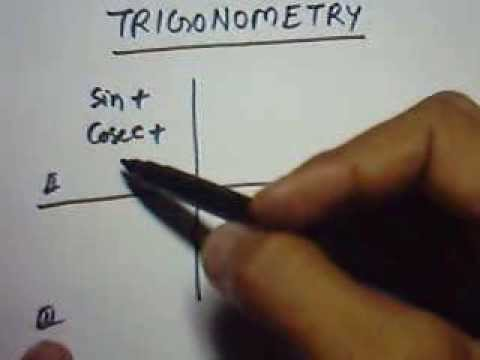## Trigonometric ratios of any angle

Subject: Optional Mathematics

#### Overview

This note includes all the information of Trigonometrical ratios of angle generalized and associated with a given angle theta.

#### Trigonometric ratios of all quadrants

Let a line OP start from OX revolve round O in the anticlockwise direction. Then it will trace positive angle XOP. If OP is in the first quadrant, then $\angle$XOP lies between 0° and 90°. If OP is in the second quadrant, then $\angle$XOP lies between 90° and 180°. Similarly, when OP is in the third or the fourth quadrant, then $\angle$XOP lies between 180° and 270° or 270° and 360° respectively.If OP revolves in the clockwise direction, the angle traced is negative.

#### Rule of signs

In the above figure, lines measured along OX' or OY' are considered positive. Lines measured along OX and OY are considered negative. If PM is perpendicular to XX', then

1. Both OM and MP are positive when OP is in the first quadrant.
2. OM is negative and MP is positive when OP is in the second quadrant.
3. Both OM and MP are negative when OP is in the third quadrant.
4. OM is positive and MP is negative when OP is in the fourth quadrant.
5. The line OP is always positive.

Thus the values of trigonometric ratios in different quadrants are as follows:-

 1st quadrant 2nd quadrant 3rd quadrant 4th quadrant all the ratios are (+e) positive sin and cosec (+ve) remaining (-ve) tan and cot (+ve) remaining (-ve) cos and sec (+ve) remaining (-v)

Ratios of (90° - A)

Let revolving line OP start from OX and trace out an angle XOP = A. Let another line OQ (= OP) revolve from OX to OYn and return back to OQ through an angle YOQ = A.

Then $\angle$XOQ = 90° - A

Draw perpendiculars PM and QN from P and Q respectively to OX.

Now, in $\triangle$s ONQ and OMP, $\angle$OQN = $\angle$MOP, $\angle$ONQ $\angle$OMP and OQ = OP. So, triangles ONQ and OMP are congruent.

Corresponding sides of a congruent triangle are equal.

$\therefore$ OQ = OP, NQ = OM and ON = MP.

Now,

sin(90° - A) = $\frac{NQ}{OQ}$= $\frac{OM}{OP}$ = cos A

cos(90° - A)= $\frac{ON}{OQ}$= $\frac{MP}{OP}$ = sin A

tan(90° - A) = $\frac{NQ}{ON}$= $\frac{OM}{MP}$ = cot A

cosec(90° - A)= $\frac{OQ}{NQ}$= $\frac{OP}{OM}$ = sec A

sec(90° - A)= $\frac{OQ}{ON}$= $\frac{OP}{MP}$ = cosec A

cot(90° - A)= $\frac{ON}{NQ}$= $\frac{MP}{OM}$ = tan A

Ratios of (90° + A)

Let a revolving line OP start from OX and trace out an angle XOP = A. Let another line OQ (=OP) revolve from OX to OY and agian to OQ through an angle YOQ = A.

Then, $\angle$XOQ = 90° + A

Draw perpendicular PM and QN from P and Q respectively to XOX'. In $\triangle$s ONQ and OMP, OQ = OP, $\angle$OQN = $\angle$MOP and $\angle$ONQ =$\angle$OMP. So, $\triangle$ ONQ ≅$\triangle$OMP.

Corresponding sides of congruent triangles are equal.

So, OQ = OP, ON = MP and NQ = OM.

By the rule of signs, OQ = OP, ON = -MP and NQ = OM.

Now,

sin(90° + A) = $\frac{NQ}{OQ}$= $\frac{OM}{OP}$ = cos A

cos(90° + A)= $\frac{ON}{OQ}$= $\frac{-MP}{OP}$ = -sin A

tan(90° + A) = $\frac{NQ}{ON}$= $\frac{OM}{-MP}$ = -cot A

cosec(90° + A)= $\frac{OQ}{NQ}$= $\frac{OP}{OM}$ = sec A

sec(90° + A)= $\frac{OQ}{ON}$= $\frac{OP}{-MP}$ = -cosec A

cot(90° + A)= $\frac{ON}{NQ}$= $\frac{-MP}{OM}$ = -tan A

Ratios of (180° - A)

Let a revolving line OP start from OX and trace out an angle XOP = A. Let another line OP (= OP) revolve from OX to OX' and return to OQ through an angle X'OQ = A.

Then, $\angle$XOQ = 180° - A.

Draw perpendicular PM and QN from P and Q respectively to XOX'.

In $\triangle$sONQ and OMP, $\angle$ONQ =$\angle$OMP,$\angle$QON =$\angle$POM and OQ = OP. So, $\triangle$ONQ≅ $\triangle$OMP.

Corresponding sides of congruent triangles are equal.

$\therefore$OQ = OP, ON = OM and NQ = MP.

By the rule of signs, OQ = OP, ON = -OM and NQ = MP

Now,

sin(180° - A) = $\frac{NQ}{OQ}$= $\frac{MP}{OP}$ =sin A

cos(180° - A)= $\frac{ON}{OQ}$= $\frac{-OM}{OP}$ = -cos A

tan(180° - A) = $\frac{NQ}{ON}$= $\frac{MP}{-OM}$ = -tan A

cosec(180° - A)= $\frac{OQ}{NQ}$= $\frac{OP}{MP}$ = cosec A

sec(180° - A)= $\frac{OQ}{ON}$= $\frac{OM}{-OM}$ = -sec A

cot(180° - A)= $\frac{ON}{NQ}$= $\frac{-OM}{MP}$ = -cot A

Ratios of (180° + A)

Let a revolving line OP start from OX and trace out an angle XOP = A. Let another line OQ (= OP) revolve from OX to OX' and again to OQ through an angle X'OQ = A.

Then, $\angle$XOQ = 180° + A.

Draw perpendiculars PM and QN from P and Q respectively to XOX'.

In $\triangle$sONQ and OMP, $\angle$ONQ =$\angle$OMP,$\angle$QON =$\angle$POM and OQ = OP.

$\therefore$ $\triangle$ONQ = $\triangle$OMP.

Corresponding sides of congruent triangles are equal.

$\therefore$ OQ = OP, ON = OM and NQ = MP.

By the rule of signs, OQ = OP, ON = -OM and NQ = -MP.

Now,

sin(180° + A) = $\frac{NQ}{OQ}$= $\frac{-OM}{OP}$ = -sin A

cos(180° + A)= $\frac{ON}{OQ}$= $\frac{-OM}{OP}$ = -cos A

tan(180° + A) = $\frac{NQ}{ON}$= $\frac{-MP}{-OM}$ = tan A

cosec(180° + A)= $\frac{OQ}{NQ}$= $\frac{OP}{-MP}$ = -cosec A

sec(180° +A)= $\frac{OQ}{ON}$= $\frac{OP}{-OM}$ = -sec A

cot(180° +A)= $\frac{ON}{NQ}$= $\frac{-OM}{-MP}$ = cot A

Ratios of (270° - A)

Let a revolving line OP start from OX and trace out an angle XOp = A. Let another line OQ = (= OP) revolve from OX to OY' and return to OQ through an angle Y'OQ = A.

Then, $\angle$XOQ = 270° - A.

Draw perpendicular PM and QN from P and Q respectively to XOX'.

In $\triangle$sONQ and OMP, $\angle$ONQ =$\angle$OMP,$\angle$OQN =$\angle$MOP ans OQ = OP.

$\therefore$ $\triangle$ONQ ≅$\triangle$OMP.

Corresponding sides of congruent triangles are equal.

So, OQ = OP, ON = MP, NQ = OM

By the rule of signs, OQ= OP, ON = -MP and NQ = -OM.

Now,

sin(270° - A) = $\frac{NQ}{OQ}$= $\frac{-OM}{OP}$ = -cos A

cos(270° -A)= $\frac{ON}{OQ}$= $\frac{-MP}{OP}$ = -sin A

tan(270° - A) = $\frac{NQ}{ON}$= $\frac{-OM}{-MP}$ = cot A

cosec(270° - A)= $\frac{OQ}{NQ}$= $\frac{OP}{-OM}$ = -sec A

sec(270° -A)= $\frac{OQ}{ON}$= $\frac{OP}{-MP}$ = -cosec A

cot(270° - A)= $\frac{ON}{NQ}$= $\frac{-MP}{-OM}$ = tan A

Ratios of (270° + A)

Let s revolving

line OP start from OX and trace out an angle XOP = A. Let another line OQ = (- OP) revolve from OX to OY' and return to OQ through an angle Y'OQ = A.

Then, $\angle$XOQ = 270° - A.

Draw perpendicular PM and QN from P and Q respectively to XOX'.

In $\triangle$sONQ and OMP, $\angle$ONQ =$\angle$OMP,$\angle$OQN =$\angle$MOP ans OQ = OP.

$\therefore$ $\triangle$ONQ ≅$\triangle$OMP.

Corresponding sides of congruent triangles are equal.

So, OQ = OP, ON = MP, NQ = OM

By the rule of signs, OQ= OP, ON = -MP and NQ = -OM.

Now,

sin(270° +A) = $\frac{NQ}{OQ}$= $\frac{-OM}{OP}$ = -cos A

cos(270° +A)= $\frac{ON}{OQ}$= $\frac{MP}{OP}$ = sin A

tan(270° +A) = $\frac{NQ}{ON}$= $\frac{-OM}{MP}$ = -cot A

cosec(270° +A)= $\frac{OQ}{NQ}$= $\frac{OP}{-OM}$ = -sec A

sec(270° + A)= $\frac{OQ}{ON}$= $\frac{OP}{MP}$ = cosec A

cot(270° +A)= $\frac{ON}{NQ}$= $\frac{MP}{-OM}$ = -tan A

Ratios of (360° - A)

Let a revolving line OP start from OX and trace out an angle XOP = A. Let another line OQ = OP revolve from OX to OX and return back to OQ through an angle XOQ = A.

Then, $\angle$XOQ = 360° - A.

Draw perpendiculars PM and QN from P and Q respectively to OX.

In $\triangle$sONQ and OMP, $\angle$ONQ = $\angle$OMP, $\angle$NOQ = $\angle$MOP and OQ = OP.

$\therefore$ $\triangle$ ONQ≅ $\triangle$ OMP.

Corresponding sides of congruent triangles are equal.

So, OQ = OP, ON = OM and NQ = MP.

By the rule of signs, OQ = OP, ON = OM and NQ = -MP.

Now,

sin(360° - A) = $\frac{NQ}{OQ}$= $\frac{-MP}{OP}$ = -sin A

cos(360° - A)= $\frac{ON}{OQ}$= $\frac{OM}{OP}$ = cos A

tan(360° - A) = $\frac{NQ}{ON}$= $\frac{-MP}{OM}$ = -tan A

cosec(360° - A)= $\frac{OQ}{NQ}$= $\frac{OP}{-MP}$ = -cosec A

sec(360° - A)= $\frac{OQ}{ON}$= $\frac{OP}{OM}$ = sec A

cot(360° - A)= $\frac{ON}{NQ}$= $\frac{OM}{-MP}$ = -cot A

Ratios of negative angle (-A)

Let a revolving line OP start from OX and trace out an angle XOP = A. Let another line OQ = (-OP) revolve from OX to OX and return back to OQ through an angle XOQ = A.

Then $\angle$XOQ = -A.

Draw perpendiculars PM and QN from P and Q respectively to OX. Then similarly, as above,

OQ = OP, ON = OM and NQ = -MP

Then,

sin(-A) = $\frac{NQ}{OQ}$= $\frac{-MP}{OP}$ = -sin A

cos(-A)= $\frac{ON}{OQ}$= $\frac{OM}{OP}$ = cos A

tan(-A) = $\frac{NQ}{ON}$= $\frac{-MP}{OM}$ = -tan A

cosec(-A)= $\frac{OQ}{NQ}$= $\frac{OP}{-MP}$ = -cosec A

sec(-A)= $\frac{OQ}{ON}$= $\frac{OP}{OM}$ = sec A

cot(-A)= $\frac{ON}{NQ}$= $\frac{OM}{-MP}$ = -cot A

##### Things to remember

Values of every trigonometric ratio in different quadrants are:

 1st quadrant 2nd quadrant 3rd quadrant 4th quadrant all the ratios are (+e) positive sin and cosec (+ve) remaining (-ve) tan and cot (+ve) remaining (-ve) cos and sec (+ve) remaining (-v)
• It includes every relationship which established among the people.
• There can be more than one community in a society. Community smaller than society.
• It is a network of social relationships which cannot see or touched.
• common interests and common objectives are not necessary for society.
##### Videos for Trigonometric ratios of any angle##### Trigonometric ratios for angles greater than 90 degrees##### Trigonometric Ratios of Acute Angles##### Questions and Answers

LHS =

= sin 9° ×  sin 27° = sin(90° - 81°)  sin(90° - 63°)

=  cos 63° × cos 81° = RHS

$\therefore$ LHS = RHS proved.

Soln

LHS = sin1°. sin2°. sin45° .sec88° .sec89° = 1

= sin1°.sin2°.1.sec(90° - 2°).sec(90° - 1°)

= sin1°.sin2°.1.cosec2°.cosec1°

= sin1°.cosec1°.sin2°.cosec2°

= 1×1

= 1 = RHS

$\therefore$ LHS = RHS

LHS = sec$\theta$.cosec$\theta$(90° - $\theta$) - tan$\theta$.cot(90° - $\theta$) = 1

= sec$\theta$.sec$\theta$ - tan$\theta$.tan$\theta$

= sec2$\theta$ - tan2$\theta$

= 1

= 1 = RHS

$\therefore$ LHS = RHS proved.

Soln

LHS = $\frac{cos(90°+θ).sec(-θ).tan(180° - θ)}{sec(360° + θ).sin(180°+θ).cot(90°-θ)}$ = -1

= $\frac{cos(90°+θ).sec(-θ).tan(180° - θ)}{sec(4×90° + θ).sin(2×90°+θ).cot(90°-θ)}$

= $\frac{-sinθ.secθ(-tanθ)}{secθ(-sinθ).tanθ}$

= -1 = RHS

$\therefore$ LHS = RHS proved.

Soln

LHS = sin70°.cos20° + sin20°.cos70°

= sin70°.cos(90° - 70°) + sin(90° - 70°).cos70°

= sin70°. sin70° + cos70°.cos70°

= sin270° + cos270° = 1